The `tf.data` API of Tensorflow is a great way to build a pipeline for sending data to the GPU. Setting up data augmentation can be a bit tricky though. In this tutorial I will go through the steps of setting up a data augmentation pipeline. The table of contents for this post:

Note: I have used Tensorflow eager in this post, but the same approach can also be used for the graph mode of Tensorflow.

## Some sample data

To illustrate the different augmentation techniques we need some demo data. CIFAR10 is available through Tensorflow so that is an easy start. For illustration purposes I take the first 8 examples of CIFAR10 and build a dataset with this. In real world scenarios this would be replaced by your own data loading logic.

``````import tensorflow as tf
tf.enable_eager_execution()

(x_train, y_train), (x_test, y_test) = tf.keras.datasets.cifar10.load_data()
dataset = tf.data.Dataset.from_tensor_slices((x_train[0:8] / 255).astype(np.float32))
``````

With a simple function we can plot images from this dataset:

``````import numpy as np
import matplotlib.pyplot as plt

def plot_images(dataset, n_images, samples_per_image):
output = np.zeros((32 * n_images, 32 * samples_per_image, 3))

row = 0
for images in dataset.repeat(samples_per_image).batch(n_images):
output[:, row*32:(row+1)*32] = np.vstack(images.numpy())
row += 1

plt.figure()
plt.imshow(output)
plt.show()
``````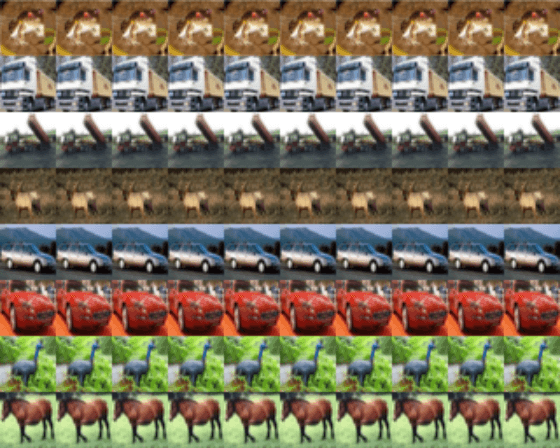## Implementing augmentations

To augment the dataset it can beneficial to make augmenter functions: a function that receives an image (a `tf.Tensor`) and returns a new augmented image. By defining functions for each augmentation operation we can easily attach them to datasets and control when they are evaluated. The augmenter functions I use are based on the following signature:

``````def augment(x: tf.Tensor) -> tf.Tensor:
"""Some augmentation

Args:
x: Image

Returns:
Augmented image
"""
x = .... # augmentation here
return x
``````

With this basic recipe for an augmenter function we can implement the augmenters itself. Here I will show examples of:

• Orientation (flipping and rotation)
• Color augmentations (hue, saturation, brightness, contrast)
• Zooming

Not all of these augmentations are necessarily applicable to CIFAR10; e.g. learning to detect flipped trucks is maybe not that beneficial for the task at hand. Nevertheless, I show them here as an example as they can be useful for tasks that are more orientation invariant. Of course, there are many more augmentations that could be useful, but most of them follow the same approach.

### Rotation and flipping

One of the most simplest augmentations is rotating the image 90 degrees. For this we can use the `rot90` function of Tensorflow. To get a new random rotation for each image we need to use a random function from Tensorflow itself. Random functions from Tensorflow are evaluated for every input, functions from numpy or basic python only once which would result in a static augmentation.

``````def rotate(x: tf.Tensor) -> tf.Tensor:
"""Rotation augmentation

Args:
x: Image

Returns:
Augmented image
"""

# Rotate 0, 90, 180, 270 degrees
return tf.image.rot90(x, tf.random_uniform(shape=[], minval=0, maxval=4, dtype=tf.int32))

``````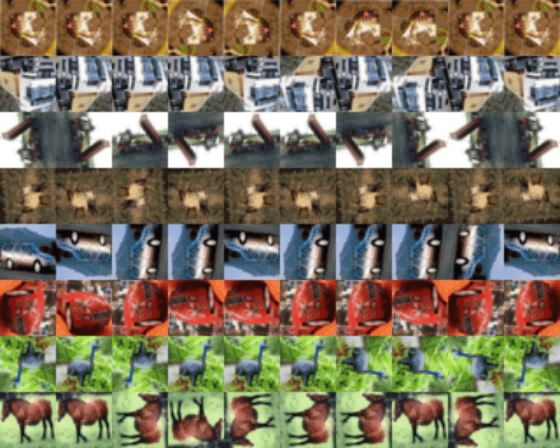Flipping is another easy-to-implement augmentation. For these augmentations we do not have to use a random number generator as Tensorflow has a built-in function that does this for us: random_flip_left_right and random_flip_up_down.

``````
def flip(x: tf.Tensor) -> tf.Tensor:
"""Flip augmentation

Args:
x: Image to flip

Returns:
Augmented image
"""
x = tf.image.random_flip_left_right(x)
x = tf.image.random_flip_up_down(x)

return x
``````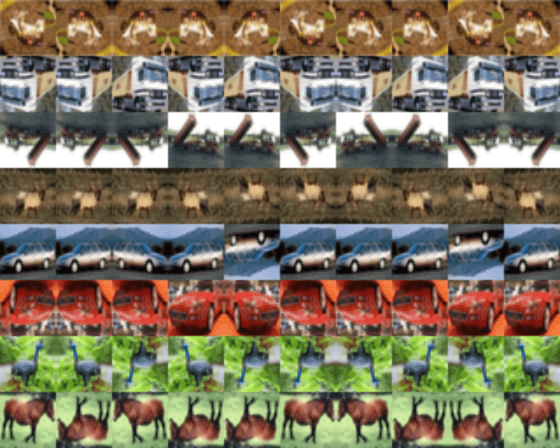### Color augmentations

Color augmentations are applicable to almost every image learning task. In Tensorflow there are four color augmentations readily available: hue, saturation, brightness and contrast. These functions only require a range and will result in an unique augmentation for each image.

``````def color(x: tf.Tensor) -> tf.Tensor:
"""Color augmentation

Args:
x: Image

Returns:
Augmented image
"""
x = tf.image.random_hue(x, 0.08)
x = tf.image.random_saturation(x, 0.6, 1.6)
x = tf.image.random_brightness(x, 0.05)
x = tf.image.random_contrast(x, 0.7, 1.3)
return x
``````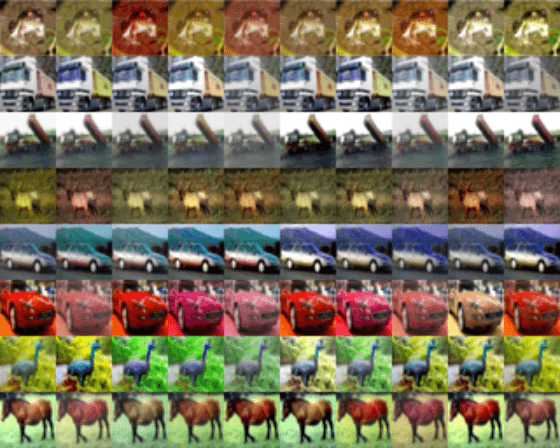### Zooming

Zooming is a powerful augmentation that can make a network robust to (small) changes in object size. This augmentation is a bit harder to implement as there is no single function that performs this operation completely. The Tensorflow function crop_and_resize function comes close as it can crop an image and then resize it to an arbitrary size. The function requires a list of ‘crop boxes’ that contain normalized coordinates (between 0 and 1) for cropping.

In the augmentation function below we first create 20 crop boxes using numpy. These boxes are created once and then passed on to the `crop_and_resize` function. This function returns a new image for each crop box, resulting in 20 potential cropped images for each input image. By using `tf.random_uniform` we can randomly select one of these crops. `tf.random_uniform` will give new random numbers during training so is safe to use here.

To make sure that some part of our data retains it original dimension, a `tf.cond` call can be used. `tf.cond` expects three parameters: a predicate (or condition), a true function `true_fn` and a false function `false_fn`. The predicate should be an operation that evaluates to true or false, after which `true_fn` or `false_fn` is called respectively. In our case we use a random number generator to return true in 50% of the calls. `true_fn` is set to the cropping function and `false_fn` to a identity function returning the original image.

Note: Do not use `np.random` functions for generating random numbers in these augmenter functions. These are only evaluated once in the TF data pipeline and will result in the same augmentation applied to all images.

``````def zoom(x: tf.Tensor) -> tf.Tensor:
"""Zoom augmentation

Args:
x: Image

Returns:
Augmented image
"""

# Generate 20 crop settings, ranging from a 1% to 20% crop.
scales = list(np.arange(0.8, 1.0, 0.01))
boxes = np.zeros((len(scales), 4))

for i, scale in enumerate(scales):
x1 = y1 = 0.5 - (0.5 * scale)
x2 = y2 = 0.5 + (0.5 * scale)
boxes[i] = [x1, y1, x2, y2]

def random_crop(img):
# Create different crops for an image
crops = tf.image.crop_and_resize([img], boxes=boxes, box_ind=np.zeros(len(scales)), crop_size=(32, 32))
# Return a random crop
return crops[tf.random_uniform(shape=[], minval=0, maxval=len(scales), dtype=tf.int32)]

choice = tf.random_uniform(shape=[], minval=0., maxval=1., dtype=tf.float32)

# Only apply cropping 50% of the time
return tf.cond(choice < 0.5, lambda: x, lambda: random_crop(x))
``````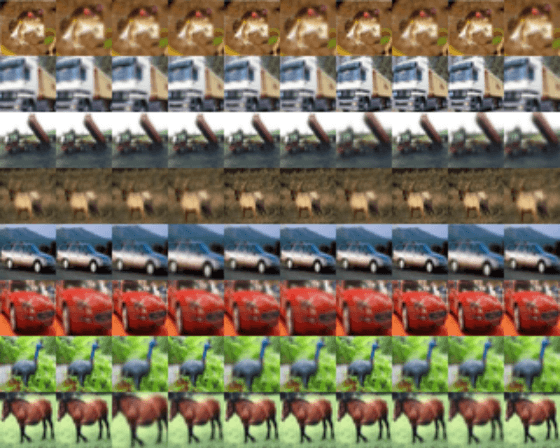## Augmenting the Dataset

With all functions defined we can combine them in to a single pipeline. Applying these functions to a Tensorflow Dataset is very easy using the `map` function. The `map` function takes a function and returns a new and augmented dataset. When this new dataset is evaluated, the data operations defined in the function will be applied to all elements in the set. Chaining map functions makes it very easy to iteratively add new data mapping operations, like augmentations.

To drastically increase the speed of these operations we can execute them in parallel, practically all Tensorflow operations support this. With the tf.Data API this is done using the `num_parallel_calls` parameter of the `map` function. When this parameter is higher than one functions will be executed in parallel. It is advised to set this parameter to the number of CPUs available.

Note: Some of these operations can result in images that have values outside the normal range of [0, 1]. To make sure that these ranges are not exceeded a clipping function such as `tf.clip_by_value` is recommended.

``````# Add augmentations
augmentations = [flip, color, zoom, rotate]

# Add the augmentations to the dataset
for f in augmentations:
# Apply the augmentation, run 4 jobs in parallel.
dataset = dataset.map(f, num_parallel_calls=4)

# Make sure that the values are still in [0, 1]
dataset = dataset.map(lambda x: tf.clip_by_value(x, 0, 1), num_parallel_calls=4)

plot_images(dataset, n_images=10, samples_per_image=15)
``````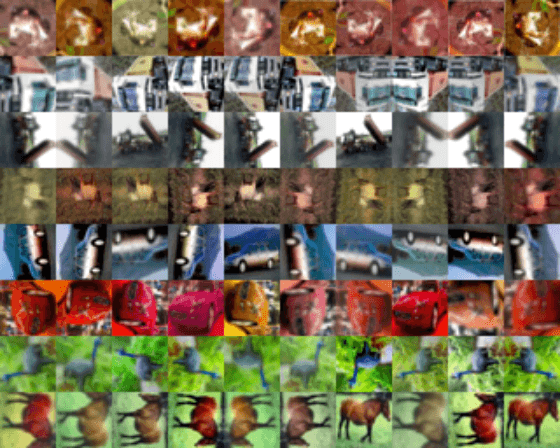If applying all augmentations is a bit to much – which it is in the example above – it is also possible to only apply them to a certain percentage of the data. For this we can use the same approach as for the zooming augmentation: a combination of a `tf.cond` and `tf.random_uniform` call.

``````for f in augmentations:
# Apply an augmentation only in 25% of the cases.
dataset = dataset.map(lambda x: tf.cond(tf.random_uniform([], 0, 1) > 0.75, lambda: f(x), lambda: x), num_parallel_calls=4)
``````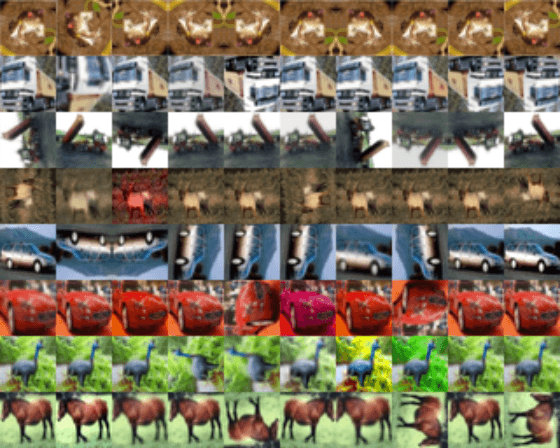That’s it! Adding more augmentations is as simple as writing a new function and adding them to the list of augmenters. Any other tips for data augmentation using the tf.Data pipeline? Let me know!

## Full code example used in this post

For convenience, all code in this post is repeated below, combined as a single script:

``````import tensorflow as tf

import numpy as np
import matplotlib.pyplot as plt

def plot_images(dataset, n_images, samples_per_image):
output = np.zeros((32 * n_images, 32 * samples_per_image, 3))

row = 0
for images in dataset.repeat(samples_per_image).batch(n_images):
output[:, row*32:(row+1)*32] = np.vstack(images.numpy())
row += 1

plt.figure()
plt.imshow(output)
plt.show()

def flip(x: tf.Tensor) -> tf.Tensor:
"""Flip augmentation

Args:
x: Image to flip

Returns:
Augmented image
"""
x = tf.image.random_flip_left_right(x)
x = tf.image.random_flip_up_down(x)

return x

def color(x: tf.Tensor) -> tf.Tensor:
"""Color augmentation

Args:
x: Image

Returns:
Augmented image
"""
x = tf.image.random_hue(x, 0.08)
x = tf.image.random_saturation(x, 0.6, 1.6)
x = tf.image.random_brightness(x, 0.05)
x = tf.image.random_contrast(x, 0.7, 1.3)
return x

def rotate(x: tf.Tensor) -> tf.Tensor:
"""Rotation augmentation

Args:
x: Image

Returns:
Augmented image
"""

return tf.image.rot90(x, tf.random_uniform(shape=[], minval=0, maxval=4, dtype=tf.int32))

def zoom(x: tf.Tensor) -> tf.Tensor:
"""Zoom augmentation

Args:
x: Image

Returns:
Augmented image
"""

# Generate 20 crop settings, ranging from a 1% to 20% crop.
scales = list(np.arange(0.8, 1.0, 0.01))
boxes = np.zeros((len(scales), 4))

for i, scale in enumerate(scales):
x1 = y1 = 0.5 - (0.5 * scale)
x2 = y2 = 0.5 + (0.5 * scale)
boxes[i] = [x1, y1, x2, y2]

def random_crop(img):
# Create different crops for an image
crops = tf.image.crop_and_resize([img], boxes=boxes, box_ind=np.zeros(len(scales)), crop_size=(32, 32))
# Return a random crop
return crops[tf.random_uniform(shape=[], minval=0, maxval=len(scales), dtype=tf.int32)]

choice = tf.random_uniform(shape=[], minval=0., maxval=1., dtype=tf.float32)

# Only apply cropping 50% of the time
return tf.cond(choice < 0.5, lambda: x, lambda: random_crop(x))

(x_train, y_train), (x_test, y_test) = tf.keras.datasets.cifar10.load_data()

data = (x_train[0:8] / 255).astype(np.float32)
dataset = tf.data.Dataset.from_tensor_slices(data)